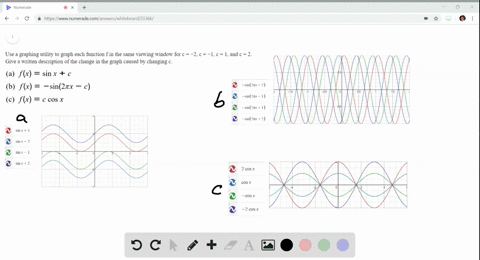🎉 The Study-to-Win Winning Ticket number has been announced! Go to your Tickets dashboard to see if you won! 🎉View Winning Ticket### Writing In Exercises 53 and $54,$ use a graphing …

04:40University of California, Berkeley
Problem 53

# Writing In Exercises 53 and $54,$ use a graphing utility to graph each function $f$ in the same viewing window for $c=-2$ , $c=-1, c=1,$ and $c=2 .$ Give a written description of the change in the graph caused by changing \$c .$$\begin{array}{l}{\text { (a) } f(x)=c \sin x} \\ {\text { (b) } f(x)=\cos (c x)} \\ {\text { (c) } f(x)=\cos (\pi x-c)}\end{array}$$

## Discussion

You must be signed in to discuss.

## Video Transcript

Okay, so these are fallen graft part one part a one C is equal to negative too. You'd have won and won. This is our graph for part B, and this is our graph part.